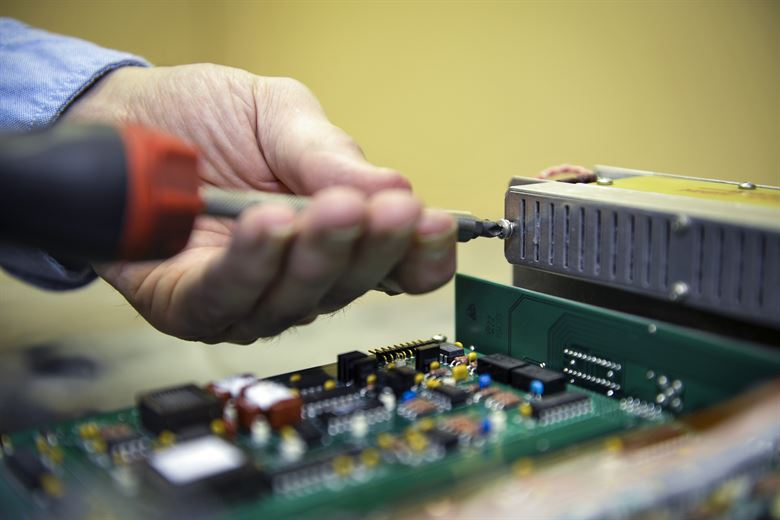Categories

# BE8255 Syllabus BASIC ELECTRICAL ELECTRONICS AND MEASUREMENT ENGINEERING Regulation 2017 Anna UniversityBE8255 Syllabus BASIC ELECTRICAL ELECTRONICS AND MEASUREMENT ENGINEERING Regulation 2017 Anna University

# BE8255 Syllabus BASIC ELECTRICAL, ELECTRONICS AND MEASUREMENT ENGINEERING

BE8255 Syllabus BASIC ELECTRICAL ELECTRONICS AND MEASUREMENT ENGINEERING Regulation 2017 Anna University Free Download. BEEME Syllabus BE8255 Free Pdf Download.

## OBJECTIVES BE8255 Syllabus BASIC ELECTRICAL ELECTRONICS AND MEASUREMENT ENGINEERING:

Understand the fundamentals of electronic circuit constructions.

To learn the fundamental laws, theorems of electrical circuits and also to analyse them

Study the basic principles of electrical machines and their performance

To study the different energy sources, protective devices and their field applications

To understand the principles and operation of measuring instruments and transducers

### UNIT I ELECTRICAL CIRCUITS ANALYSIS BE8255 Syllabus BASIC ELECTRICAL ELECTRONICS AND MEASUREMENT ENGINEERING

Ohms Law, Kirchhoff’s Law-Instantaneous power- series and parallel circuit analysis with resistive, capacitive and inductive network – nodal analysis, mesh analysis- network theorems – Thevenins theorem, Norton theorem, maximum power transfer theorem and superposition theorem, three phase supply-Instantaneous, Reactive and apparent power-star delta conversion.

#### UNIT II ELECTRICAL MACHINES BE8255 Syllabus BASIC ELECTRICAL ELECTRONICS AND MEASUREMENT ENGINEERING

DC and AC ROTATING MACHINES:Types, Construction, principle, Emf and torque equation, application Speed Control- Basics of Stepper Motor – Brushless DC motors- Transformers-Introduction- types and construction, working principle of Ideal transformer-Emf equation- All day efficiency calculation.

##### UNIT III UTILIZATION OF ELECTRICAL POWER BE8255 Syllabus BASIC ELECTRICAL ELECTRONICS AND MEASUREMENT ENGINEERING

Renewable energy sources-wind and solar panels. Illumination by lamps- Sodium Vapour, Mercury vapour, Fluorescent tube. Domestic refrigerator and air conditioner-Electric circuit, construction and working principle. Batteries-NiCd, Pb Acid and Li ion–Charge and Discharge Characteristics. Protection-need for earthing, fuses and circuit breakers.Energy Tariff calculation for domestic loads.

###### UNIT IV ELECTRONIC CIRCUITS BE8255 Syllabus BASIC ELECTRICAL ELECTRONICS AND MEASUREMENT ENGINEERING

PN Junction-VI Characteristics of Diode, zener diode, Transistors configurations – amplifiers. Op amps- Amplifiers, oscillator,rectifiers, differentiator, integrator, ADC, DAC. Multi vibrator using 555 Timer IC . Voltage regulator IC using LM 723,LM 317.

###### UNIT V ELECTRICAL MEASUREMENT BE8255 Syllabus BASIC ELECTRICAL ELECTRONICS AND MEASUREMENT ENGINEERING

Characteristic of measurement-errors in measurement, torque in indicating instruments- moving coil and moving iron meters, Energy meter and watt meter. Transducers- classification-thermo electric, RTD, Strain gauge, LVDT, LDR and piezoelectric. Oscilloscope-CRO.

TOTAL :45 PERIODS

###### OUTCOMES: BE8255 Syllabus BASIC ELECTRICAL ELECTRONICS AND MEASUREMENT ENGINEERING

Upon completion of the course, the students will be able to:

• Discuss the essentials of electric circuits and analysis.
• Discuss the basic operation of electric machines and transformers
• Introduction of renewable sources and common domestic loads.
• To understand the fundamentals of electronic circuit constructions.
• Introduction to measurement and metering for electric circuits.
 Subject Name BASIC ELECTRICAL ELECTRONICS AND MEASUREMENT ENGINEERING Subject Code BE8255 Regulation 2017 File PDF

BE8255 Important questions Basic Electrical Electronics and Measurement Engineering

BE8255 Notes Basic Electrical Electronics and Measurement Engineering

BE8255 Question Bank Basic Electrical Electronics and Measurement Engineering

Search In for BE8255 Syllabus:SAM RICHARDsays: